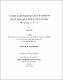## A study of the sensitivity of the H dibaryon search experiment E813 at BNL through ([Sigma]S-,p)[subscript]a[subscript]t[subscript]o[subscript]m -&gt; [Lambda] + nThis thesis is a study of the sensitivity of BNL experiment E813. Experiment E813 was a search for the H-Dibaryon (a six-quark uuddss state) at Brookhaven National Laboratory's 2-GeV/c beam line. It was insensitive to H decay modes and lifetime, and looked directly for H formation through a three step reaction mechanism. A liquid hydrogen target was used to produce $\Xi\sp-$ hyperons via the reaction $K\sp- + p \to K\sp+ +\Xi\sp-$. These $\Xi\sp-$'s were then slowed down in tungsten degraders so that a small fraction of them would stop in a liquid deuterium target located above the liquid hydrogen target to form a ($\Xi\sp-, d)\sb{atom}$. The signature for H formation via ($\Xi\sp-, d)\sb{atom}\to H + n$ would be a monoenergetic neutron in coincidence with the formation of a ($\Xi\sp-, d)\sb{atom}$. The most critical property of the experimental apparatus is the product of the hyperon stopping efficiency and the neutron detection efficiency, which is needed to quantitatively estimate the upper limit on the H formation branching ratio and to interpret the experimental results for the H particle search. This combined efficiency was calibrated with a similar three step reaction mechanism through a study of $\Sigma\sp-$ capture on hydrogen followed by ($\Sigma\sp-, p)\sb{atom}\to\Lambda + n$. The details of the calibration are discussed in this thesis.